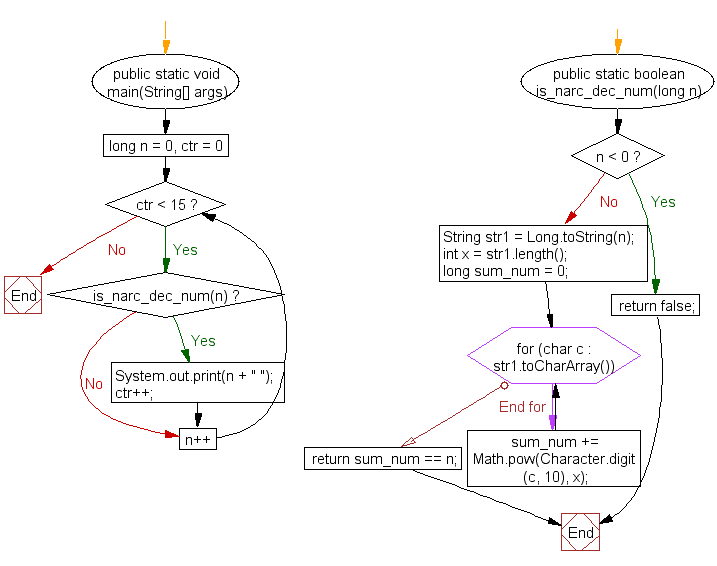﻿ Java exercises: Generate and show the first 15 narcissistic decimal numbers - w3resource# Java Exercises: Generate and show the first 15 narcissistic decimal numbers

## Java Numbers: Exercise-6 with Solution

Write a Java program to generate and show the first 15 narcissistic decimal numbers.

A Narcissistic decimal number is a non-negative integer, n that is equal to the sum of the m-th powers of each of the digits in the decimal representation of n, where m is the number of digits in the decimal representation of n.

• if n is 153
• then m , (the number of decimal digits) is 3
• we have 13 + 53 + 33 = 1 + 125 + 27 = 153
• and so 153 is a narcissistic decimal number .

Narcissistic numbers in various bases :
The sequence of base 10 narcissistic numbers starts: 0, 1, 2, 3, 4, 5, 6, 7, 8, 9, 153, 370, ……
The sequence of base 8 narcissistic numbers starts: 0, 1, 2, 3, 4, 5, 6, 7, 24, 64, 134, 205,….

Pictorial Presentation:Sample Solution:

Java Code:

``````// https://rosettacode.org/
public class Example6 {
public static void main(String[] args){
for(long n = 0, ctr = 0; ctr < 15; n++){
if(is_narc_dec_num(n)){
System.out.print(n + " ");
ctr++;
}
}
System.out.println();
}
public static boolean is_narc_dec_num(long n){
if(n < 0) return false;

String str1 = Long.toString(n);
int x = str1.length();
long sum_num = 0;

for(char c : str1.toCharArray()){
sum_num += Math.pow(Character.digit(c, 10), x);
}
return sum_num == n;
}

}
```
```

Sample Output:

```0 1 2 3 4 5 6 7 8 9 153 370 371 407 1634
```

Flowchart:Java Code Editor:

What is the difficulty level of this exercise?

﻿

## Java: Tips of the Day

Array vs ArrayLists:

The main difference between these two is that an Array is of fixed size so once you have created an Array you cannot change it but the ArrayList is not of fixed size. You can create instances of ArrayLists without specifying its size. So if you create such instances of an ArrayList without specifying its size Java will create an instance of an ArrayList of default size.

Once an ArrayList is full it re-sizes itself. In fact, an ArrayList is internally supported by an array. So when an ArrayList is resized it will slow down its performance a bit as the contents of the old Array must be copied to a new Array.

At the same time, it's compulsory to specify the size of an Array directly or indirectly while creating it. And also Arrays can store both primitives and objects while ArrayLists only can store objects.

Ref: https://bit.ly/3o8L2KH